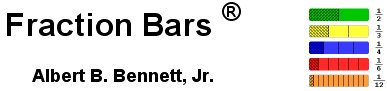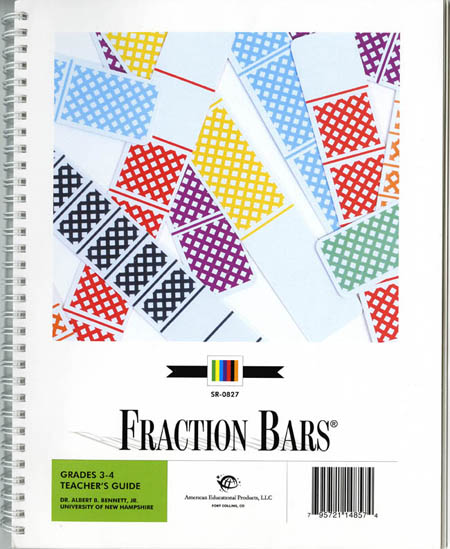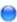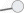Fraction Bars ProgramsHome > Products > Fraction Bars Teacher's Guide Grades 3-4

 Fraction Bars Teacher's Guide Grades 3-4- NEW FULL COLOR Grades 3-4 Fraction Bars Teacher's Guide contains complete teaching information for fractions at these grade levels. The lessons contain instruction for the following topics: basic concept of fractions equality and inequality of fractions relationship of fractions decimals and percents introduction to addition and subtraction of fractions measuring with number lines and rulers mixed numbers rounding multiplication of fractions times fractions and an introduction to probability. These lessons are followed by masters that are designed for students to complete in class. All program components such as Fraction Bars Fraction Playing Cards games activity mats and worksheets are referenced at point-of-use in each lesson. Purchase this product from the following companies:Quality Math Products - Product Number SR0827Hand 2 Mind - Product Number IN79435Nasco - Product Number TB25371T Detail image